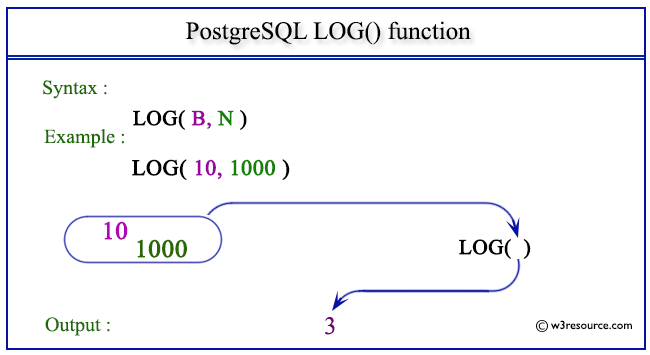# PostgreSQL LOG() function

## LOG() function

The PostgreSQL log() function is used to return the base 10 logarithm of a given number or logarithm of a number for a particular base, as specified in the argument.

Syntax:

```log()
```

PostgreSQL Version: 9.3

Pictorial presentation of PostgreSQL LOG() functionExample 1: PostgreSQL LOG() function

Code:

``````SELECT LOG(200.0) AS "Base 10 Logarithm";
```
```

Sample Output:

``` Base 10 Logarithm
--------------------
2.3010299956639812
(1 row)
```

Example 2: PostgreSQL LOG() function

Code:

``````SELECT LOG(2.0,16) AS "Base 2 Logarithm";
```
```

Sample Output:

```  Base 2 Logarithm
--------------------
4.0000000000000000
(1 row)
```

Previous: LN function
Next: MOD function

﻿